Definition

Gradient descent is a general approach used in first-order iterative optimization algorithms whose goal is to find the (approximate) minimum of a function of multiple variables. The idea is that, at each stage of the iteration, we move in the direction of the negative of the gradient vector (or computational approximation to the gradient vector). The step size that we choose depends on the exact algorithm used, and typically involves some sort of line search.

Other names for gradient descent are steepest descent and method of steepest descent.

The corresponding method, if applied to finding maxima, would move in a positive direction along the gradient vector, and is called gradient ascent. The methods are computationally equivalent: gradient descent for finding the minimum of$f$ is equivalent to gradient ascent for finding the maximum of$-f$ over the same domain.

Iterative step

Note that we use parenthesized superscripts to denote iterates and iteration-specific variables, and subscripts to denote coordinates. Parenthesized superscripts do not denote exponents. At one place below, we use a non-parenthesized superscript for an exponent, as is clear from context.

Suppose we are applying gradient descent to minimize a function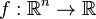$f:\R^n \to \R$. We will denote the input to$f$ as a vector$\vec{x} = (x_1,x_2,\dots,x_n)$. Given a guess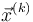$\vec{x}^{(k)}$, gradient descent computes the next guess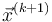$\vec{x}^{(k+1)}$ as follows:$\vec{x}^{(k+1)} = \vec{x}^{(k)} - \alpha^{(k)} \nabla f\left(\vec{x}^{(k)}\right)$

Note that the quantity$\alpha^{(k)}$ (called the learning rate) needs to be specified, and the method of choosing this constant describes the type of gradient descent. For the simplest type of gradient descent, called gradient descent with constant learning rate, all the$\alpha^{(k)}$ equal a constant$\alpha$ and are independent of the current iterate.

One aspect that distinguishes gradient descent from some variants (such as parallel coordinate descent, sequential coordinate descent, and accelerated gradient methods) is that in every iteration, we move strictly along the direction of the gradient vector, i.e.,$\vec{x}^{(k)} - \vec{x}^{(k+1)}$ is a scalar multiple of the gradient vector at$\vec{x}^{(k)}$.

Intuition behind choice of learning rate

Essentially, the learning rate should be chosen to be the reciprocal of the second derivative (often referred to as the curvature although it is not the same as curvature as used in differential geometry). There are, however, a number of subtleties. The cases are discussed below, in roughly increasing order of complexity:

Case Discussion of gradient descent with constant learning rate Variation in second derivative when restricted to a line Variation in second derivative between directions Explanation
Quadratic function of one variable Gradient descent with constant learning rate for a quadratic function of one variable Constant Constant (only one dimension, so no scope for variation) The first derivative is a linear function with slope equal to the second derivative. We need to move by the value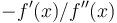$-f'(x)/f''(x)$, where$f''(x)$ is the slope of the line. The ideal learning rate is therefore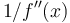$1/f''(x)$. Note that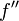$f''$ is constant.
Quadratic function of multiple variables Gradient descent with constant learning rate for a quadratic function of multiple variables Constant Variable The main challenge arises from the "elliptical geometry", i.e., the second derivative being radically different in different directions. We can get a mathematical handle of this by finding the eigenvalues of the Hessian. The ratio of the largest to the smallest eigenvalue, also known as the condition number, helps us understand the difficulty of the problem. Basically, if we are choosing a constant learning rate, it has to be chosen based on the largest eigenvalue (the$L^2$-norm) but that would result in very slow convergence along the directions of the smaller eigenvalues.
Convex function of one variable Gradient descent with constant learning rate for a convex function of one variable Variable Constant (only one dimension, so no scope for variation) The details depend on whether there is a global bound on the second derivative. If there is such a bound, and we are aware of it in advance, the quality of convergence depends on how widely the second derivative at the optimum differs from the global bound. The quadratic case is extreme: these values coincide, so we can get one-step convergence if we know the second derivative.
Convex function of multiple variables Gradient descent with constant learning rate for a convex function of multiple variables Variable Variable Two sources of variation enter the picture: the elliptical geometry arising from different second derivatives in different directions, and the ratio of the second derivative at the optimum to the global bound on the second derivative.

Corresponding root-finding method

Gradient descent is the optimization method corresponding to the slope-based root-finding method applied to the gradient vector as a vector-valued function.

Name Verbal description Is the rule stationary or dependent on number of iterations? Mathematical formalism Further comments
Gradient descent with constant learning rate (default meaning of gradient descent) Here, the step size is a fixed multiple of the gradient vector. The multiple used is termed the "learning rate" of the algorithm. The choice of learning rate affects the convergence behavior of the gradient descent. Stationary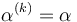$\alpha^{(k)} = \alpha$ for all$k$. The constant$\alpha$ is termed the learning rate of the algorithm. Note that this need not converge instantaneously even in the case of a quadratic function of one variable, though that is arguably the simplest nontrivial functional form to optimize. For more, see gradient descent with constant learning rate for a quadratic function of one variable. In general, if we have a global bound on the "curvature" (understood technically as the spectral norm of the Hessian), then we can take a constant learning rate that is less than or equal to the reciprocal of this bound.
Gradient descent with decaying learning rate Here, the learning rate decays. Dependent on number of iterations$\alpha^{(k)}$ depends on$k$ and approaches 0 as$k \to \infty$. A decaying learning rate results in substantially slower convergence, and is not useful when we have a global bound on the curvature, because in that case we could just choose a constant learning rate equal to the reciprocal of that global bound. A decaying learning rate is helpful in cases where the curvature is unbounded or where we don't know any bound on the curvature.
Gradient descent with exact line search The step size in each step is determined using an exact line search along the line of the gradient vector through the current point. Stationary No explicit functional form for$\alpha^{(k)}$, since it depends on details about the function. Note that this requires computation of the exact functional form restricted to the line as well as a procedure to optimize that function when restricted to that line. Neither of these may be available.
Gradient descent using Newton's method Here, the step size in each step is determined by applying one iteration (or a fixed number of iterations) of Newton's method for optimization of a function of one variable when performing the line search to determine the step size. Stationary$\alpha^{(k)}$ is the reciprocal of the second-order directional derivative of$f$ at$\vec{x}^{(k)}$ along the direction of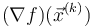$(\nabla f)(\vec{x}^{(k)})$. Explicitly, if$H$ is the Hessian of$f$ at$\vec{x}^{(k)}$, and$\vec{v} = (\nabla f)(\vec{x}^{(k)})$, then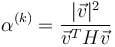$\alpha^{(k)} = \frac{|\vec{v}|^2}{\vec{v}^TH\vec{v}}$. Note that this coincides with exact line search when the function involved is a quadratic function of multiple variables, because the restriction to the line is a quadratic function, and Newton's method for optimization converges in one step for a quadratic function of one variable. Note that this requires the computation of the second derivative at the point.
Note that this method is completely different from Newton's method for optimization of a function of multiple variables, which does not move along the gradient direction, but rather, adjusts it by the inverse of the Hessian matrix.

Gradient descent in a typical machine learning context

The mathematical form of gradient descent in machine learning problems is more specific: the function that we are trying to optimize is expressible as a sum, with all the additive components having the same functional form but with different parameters (note that the parameters referred to here are the feature values for examples, not the "parameter vector" -- which plays the role of the input to the function in this case). Explicitly, the function$f$ on which we are doing gradient descent has the form:$f(\vec{x}) = \sum_{i=1}^m g(\vec{x}, \vec{v}^{(i)})$

where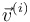$\vec{v}^{(i)}$ is a vector storing the$i^{th}$ example, and$m$ is the number of examples. In such an implementation, gradient descent can be parallelized. We have that the gradient is a sum of the gradients of each of the additive components: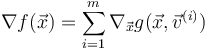$\nabla f(\vec{x}) = \sum_{i=1}^m \nabla_{\vec{x}} g(\vec{x}, \vec{v}^{(i)})$

The computation of the summands can be carried out in parallel by different processors, each of which has access to the corresponding set of$\vec{v}^{(i)}$s.